# 五、通过形态学运算转换图像

• 使用形态学过滤器腐蚀和膨胀图像
• 使用形态过滤器开放和闭合图像
• 使用形态过滤器检测边缘和角点
• 使用分水岭分割图像
• 用 GrabCut 算法提取前景对象

# 使用形态过滤器腐蚀和膨胀图像

## 准备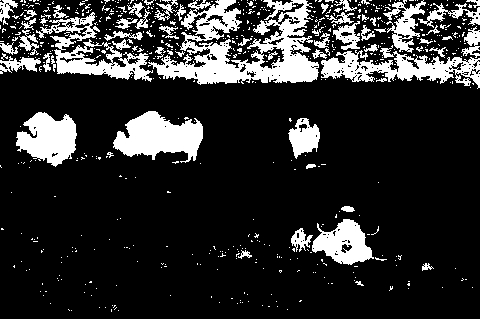## 操作步骤

   // Read input image

// Erode the image
cv::Mat eroded;  // the destination image
cv::erode(image,eroded,cv::Mat());

// Display the eroded image
cv::namedWindow("Eroded Image");");
cv::imshow("Eroded Image",eroded);

// Dilate the image
cv::Mat dilated;  // the destination image
cv::dilate(image,dilated,cv::Mat());

// Display the dilated image
cv::namedWindow("Dilated Image");
cv::imshow("Dilated Image",dilated);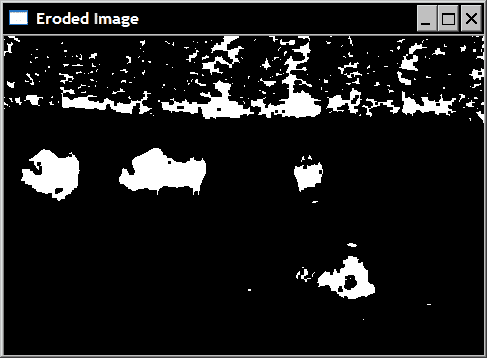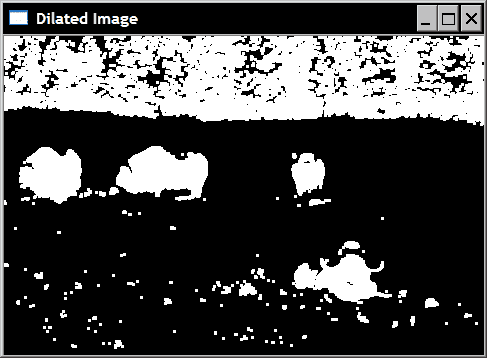## 工作原理

    cv::Mat element(7,7,CV_8U,cv::Scalar(1));
cv::erode(image,eroded,element);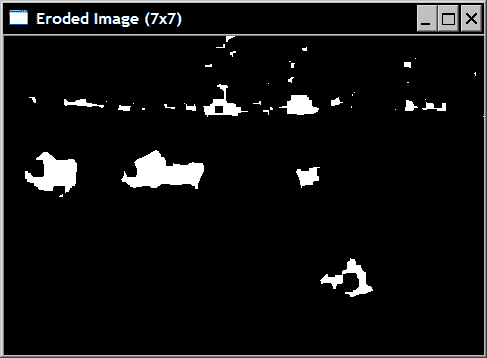// Erode the image 3 times.
cv::erode(image,eroded,cv::Mat(),cv::Point(-1,-1),3);


• 图像的侵蚀等同于补充图像的膨胀的补充。
• 图像的膨胀等效于补充图像的侵蚀的补充。

## 更多

   cv::erode(image,image,cv::Mat());


OpenCV 为您创建所需的临时映像，以使其正常工作。

# 使用形态过滤器开放和闭合图像

## 操作步骤

   cv::Mat element5(5,5,CV_8U,cv::Scalar(1));
cv::Mat closed;
cv::morphologyEx(image,closed,cv::MORPH_CLOSE,element5);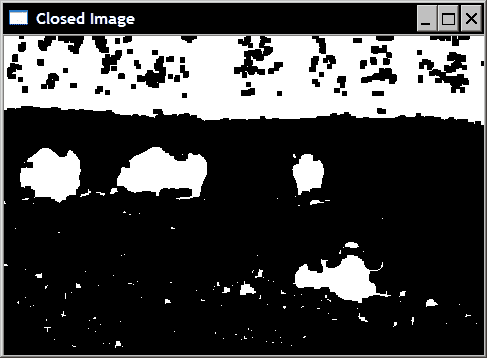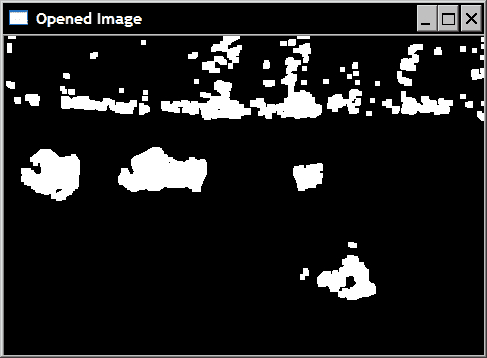cv::Mat opened;
cv::morphologyEx(image,opened,cv::MORPH_OPEN,element5);


## 工作原理

• 闭合被定义为图像膨胀的腐蚀。
• 开放被定义为图像腐蚀的膨胀。

   // dilate original image
cv::dilate(image,result,cv::Mat());
// in-place erosion of the dilated image
cv::erode(result,result,cv::Mat());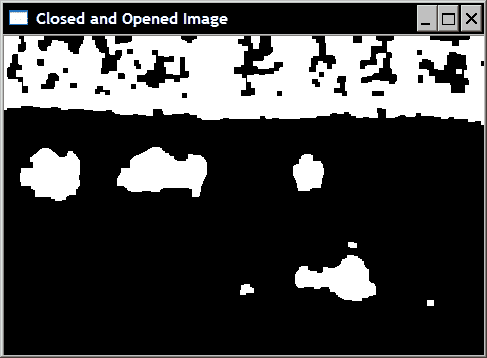# 使用形态过滤器检测边缘和角点

## 入门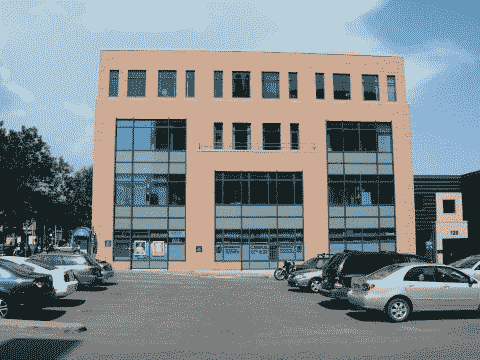## 操作步骤

class MorphoFeatures {

private:

// threshold to produce binary image
int threshold;
// structuring elements used in corner detection
cv::Mat cross;
cv::Mat diamond;
cv::Mat square;
cv::Mat x;


cv::Mat getEdges(const cv::Mat &image) {

cv::Mat result;
cv::morphologyEx(image,result,

// Apply threshold to obtain a binary image
applyThreshold(result);

return result;
}


void applyThreshold(cv::Mat& result) {

// Apply threshold on result
if (threshold>0)
cv::threshold(result, result,
threshold, 255, cv::THRESH_BINARY);
}


// Create the morphological features instance
MorphoFeatures morpho;
morpho.setThreshold(40);

// Get the edges
cv::Mat edges;
edges= morpho.getEdges(image);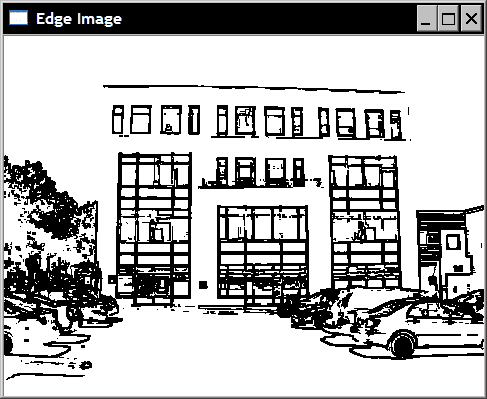MorphoFeatures() : threshold(-1),
cross(5,5,CV_8U,cv::Scalar(0)),
diamond(5,5,CV_8U,cv::Scalar(1)),
square(5,5,CV_8U,cv::Scalar(1)),
x(5,5,CV_8U,cv::Scalar(0)){

// Creating the cross-shaped structuring element
for (int i=0; i<5; i++) {

cross.at<uchar>(2,i)= 1;
cross.at<uchar>(i,2)= 1;
}

// Creating the diamond-shaped structuring element
diamond.at<uchar>(0,0)= 0;
diamond.at<uchar>(0,1)= 0;
diamond.at<uchar>(1,0)= 0;
diamond.at<uchar>(4,4)= 0;
diamond.at<uchar>(3,4)= 0;
diamond.at<uchar>(4,3)= 0;
diamond.at<uchar>(4,0)= 0;
diamond.at<uchar>(4,1)= 0;
diamond.at<uchar>(3,0)= 0;
diamond.at<uchar>(0,4)= 0;
diamond.at<uchar>(0,3)= 0;
diamond.at<uchar>(1,4)= 0;

// Creating the x-shaped structuring element
for (int i=0; i<5; i++) {

x.at<uchar>(i,i)= 1;
x.at<uchar>(4-i,i)= 1;
}
}


cv::Mat getCorners(const cv::Mat &image) {

cv::Mat result;

// Dilate with a cross
cv::dilate(image,result,cross);

// Erode with a diamond
cv::erode(result,result,diamond);

cv::Mat result2;
// Dilate with a X
cv::dilate(image,result2,x);

// Erode with a square
cv::erode(result2,result2,square);

// Corners are obtained by differencing
// the two closed images
cv::absdiff(result2,result,result);

// Apply threshold to obtain a binary image
applyThreshold(result);

return result;
}


void drawOnImage(const cv::Mat& binary,
cv::Mat& image) {

cv::Mat_<uchar>::const_iterator it=
binary.begin<uchar>();
cv::Mat_<uchar>::const_iterator itend=
binary.end<uchar>();

// for each pixel
for (int i=0; it!= itend; ++it,++i) {
if (!*it)
cv::circle(image,
cv::Point(i%image.step,i/image.step),
5,cv::Scalar(255,0,0));
}
}


// Get the corners
cv::Mat corners;
corners= morpho.getCorners(image);

// Display the corner on the image
morpho.drawOnImage(corners,image);
cv::namedWindow("Corners on Image");
cv::imshow("Corners on Image",image);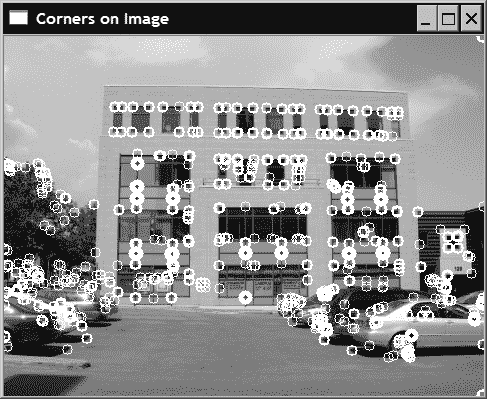## 工作原理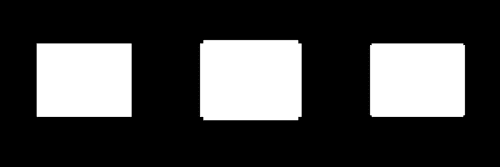## 另见

The article, Morphological gradients by J.-F. Rivest, P. Soille, S. Beucher, ISET's symposium on electronic imaging science and technology, SPIE, Feb. 1992, for more on morphological gradient.

The article A modified regulated morphological corner detector by F.Y. Shih, C.-F. Chuang, V. Gaddipati, Pattern Recognition Letters , volume 26, issue 7, May 2005, for more information on morphological corner detection.


# 使用分水岭分割图像

## 操作步骤

class WatershedSegmenter {

private:

cv::Mat markers;

public:

void setMarkers(const cv::Mat& markerImage) {

// Convert to image of ints
markerImage.convertTo(markers,CV_32S);
}

cv::Mat process(const cv::Mat &image) {

// Apply watershed
cv::watershed(image,markers);

return markers;
}


   // Eliminate noise and smaller objects
cv::Mat fg;
cv::erode(binary,fg,cv::Mat(),cv::Point(-1,-1),6);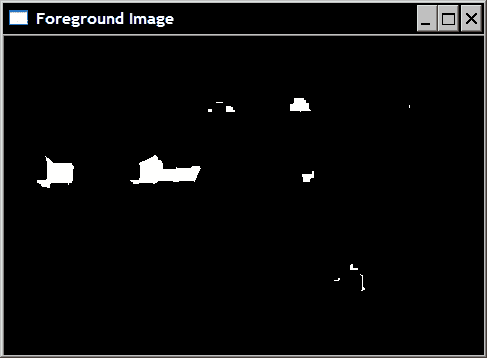// Identify image pixels without objects
cv::Mat bg;
cv::dilate(binary,bg,cv::Mat(),cv::Point(-1,-1),6);
cv::threshold(bg,bg,1,128,cv::THRESH_BINARY_INV);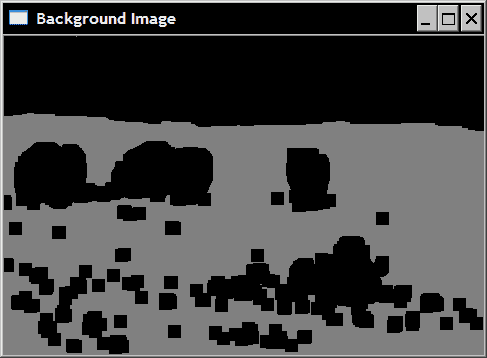// Create markers image
cv::Mat markers(binary.size(),CV_8U,cv::Scalar(0));
markers= fg+bg;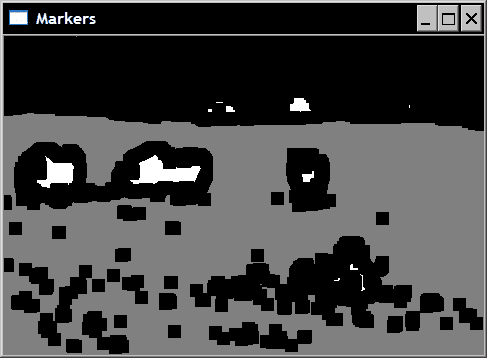// Create watershed segmentation object
WatershedSegmenter segmenter;

// Set markers and process
segmenter.setMarkers(markers);
segmenter.process(image);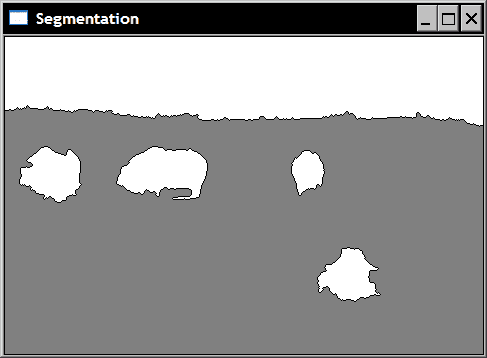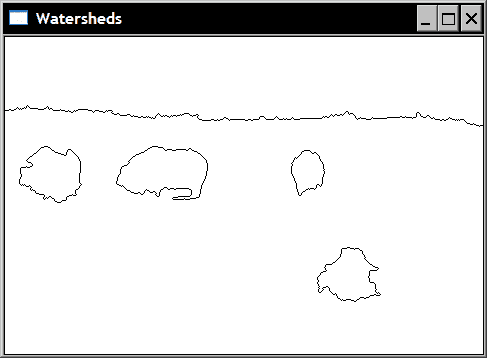## 工作原理

     // Return result in the form of an image
cv::Mat getSegmentation() {

cv::Mat tmp;
// all segment with label higher than 255
// will be assigned value 255
markers.convertTo(tmp,CV_8U);

return tmp;
}


     // Return watershed in the form of an image
cv::Mat getWatersheds() {

cv::Mat tmp;
// Each pixel p is transformed into
// 255p+255 before conversion
markers.convertTo(tmp,CV_8U,255,255);

return tmp;
}


## 另见

The article The viscous watershed transform by C. Vachier, F. Meyer, Journal of Mathematical Imaging and Vision, volume 22, issue 2-3, May 2005, for more information on the watershed transform.


# 使用 GrabCut 算法提取前景对象

OpenCV 提出了另一种流行的图像分割算法：GrabCut 算法的实现。 该算法不是基于数学形态学的，但是我们在这里介绍它，因为它显示了与前面秘籍中提出的分水岭分割算法的一些相似之处。 GrabCut 在计算上比分水岭贵，但通常可以产生更准确的结果。 当一个人想要在静止图像中提取前景对象（例如，将一个对象从一张图片剪切并粘贴到另一张图片）时，这是最好的算法。

## 操作步骤

cv::grabCut函数易于使用。 您只需要输入图像并将其某些像素标记为属于背景或前景。 基于此部分标记，该算法将确定完整图像的前景/背景分割。

   // Open image

// define bounding rectangle
// the pixels outside this rectangle
// will be labeled as background
cv::Rect rectangle(10,100,380,180);


   cv::Mat result; // segmentation (4 possible values)
cv::Mat bgModel,fgModel; // the models (internally used)
// GrabCut segmentation
cv::grabCut(image,    // input image
result,      // segmentation result
rectangle,   // rectangle containing foreground
bgModel,fgModel, // models
5,           // number of iterations
cv::GC_INIT_WITH_RECT); // use rectangle


• cv::GC_BGD，用于肯定属于背景的像素（例如，在我们的示例中，矩形外部的像素）
• cv::GC_FGD，用于肯定属于前景的像素（在我们的示例中没有）
• cv::GC_PR_BGD，用于可能属于背景的像素
• cv::GC_PR_FGD用于可能属于前景的像素（在我们的示例中为矩形内像素的初始值）。

   // Get the pixels marked as likely foreground
cv::compare(result,cv::GC_PR_FGD,result,cv::CMP_EQ);
// Generate output image
cv::Mat foreground(image.size(),CV_8UC3,
cv::Scalar(255,255,255));
image.copyTo(foreground,// bg pixels are not copied
result);


   // checking first bit with bitwise-and
result= result&1; // will be 1 if FG


   // Generate output image
cv::Mat foreground(image.size(),CV_8UC3,
cv::Scalar(255,255,255)); // all white image
image.copyTo(foreground,result); // bg pixels not copied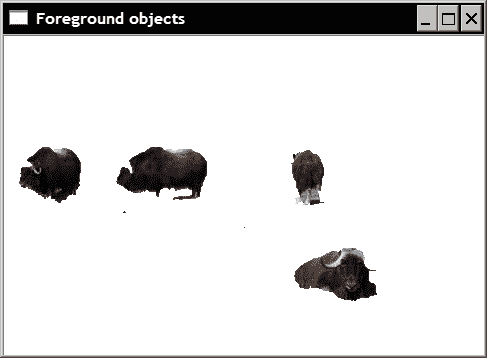## 另见

The article by C. Rother, V. Kolmogorov and A. Blake, GrabCut: Interactive Foreground Extraction using Iterated Graph Cuts in ACM Transactions on Graphics (SIGGRAPH) volume 23, issue 3, August 2004, that describes in detail the GrabCut algorithm.